Function Repository Resource:

# MetamathImport

Import and work with a Metamath database

Contributed by: Mario Carneiro and Nikolay Murzin
 ResourceFunction["MetamathImport"][src] imports Metamath source code from src and returns a MetamathObject.

## Details and Options

The src can be a File, URL or String.
Options for ResourceFunction["MetamathImport"] may be one of the following:
 "Verify" False whether to verify theorem proofs or not
MetamathObject has the following properties:
 "Symbols" list of symbols "Constants" list of constants "Variables" list of variables "Statements" list of statements "Axioms" list of axioms "Theorems" list of theorems "Definitions" list of definitions "DependencyGraph" theorem proof dependency graph {"SyntaxTree", stmt} syntax breakdown tree of a statement {"SyntaxDependencyTree", stmt} syntax dependency tree of a statement {"SimpleSyntaxTree", stmt} simplified syntax tree {"ProofTree", thm} proof tree of a theorem {"ProofGraph", thm} proof graph of a theorem {"TokenEventGraph", thm} token event proof graph of a theorem
ResourceFunction["MetamathImport"] special inputs:
 "SetMM" setmm database "NormMegillWolframAxioms" Norm Megill's proofs of the Wolfram Axioms "ASCIITable" ASCII replacement rules

## Examples

### Basic Examples (6)

Import Metamath source code with several constants and a simple axiom:

 In:=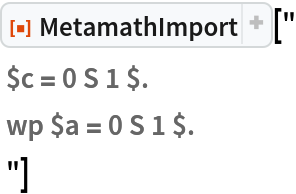Out=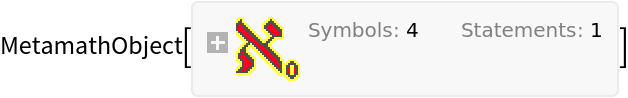Import a basic Metamath source code sample with 4 constants, 5 variables, 5 floating hypotheses, 1 axiom and 2 proved theorems:

 In:=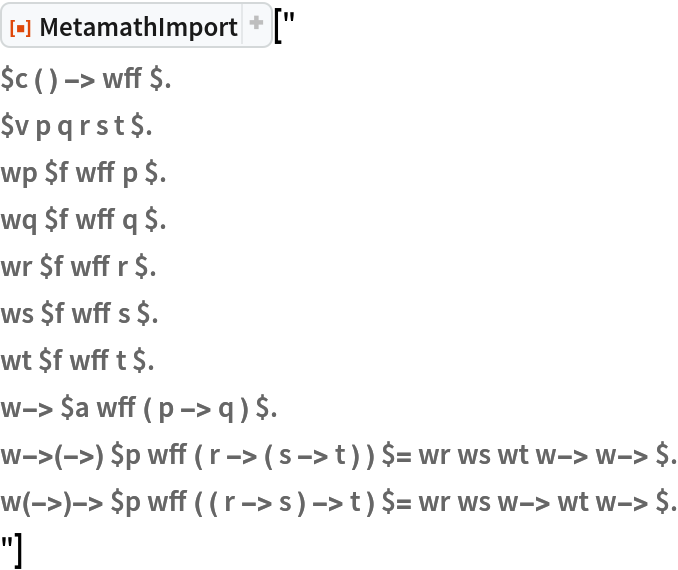Out=Construct a proof tree:

 In:=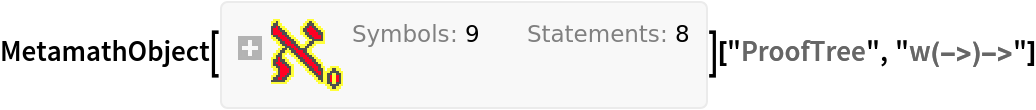Out=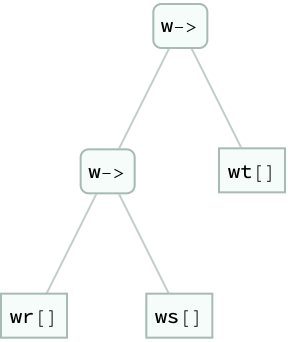Construct a proof graph:

 In:=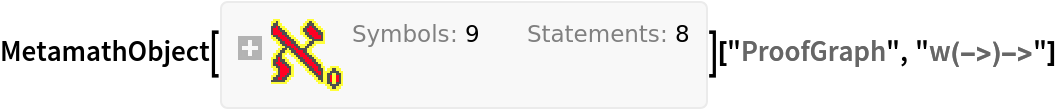Out=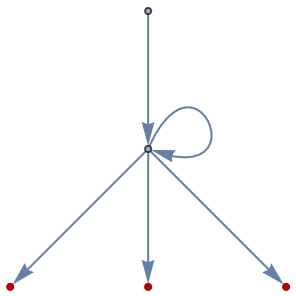Construct a detailed syntax tree breakdown:

 In:=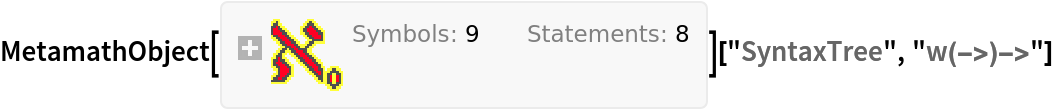Out=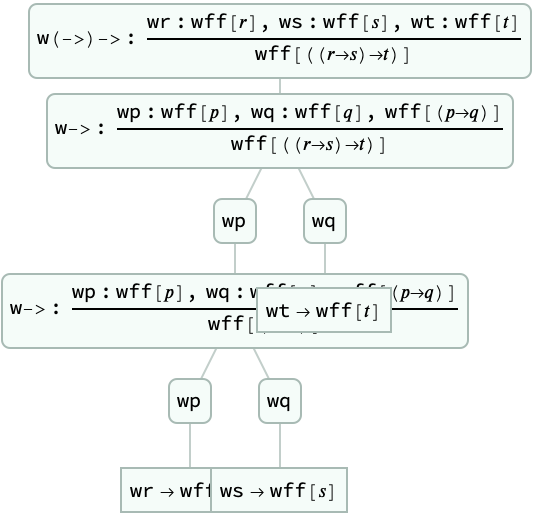Construct a simplified syntax tree:

 In:=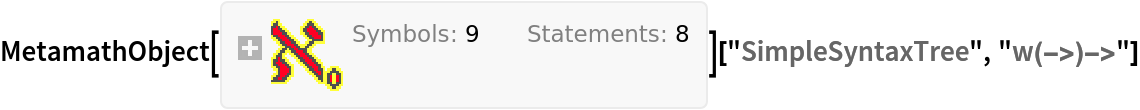Out=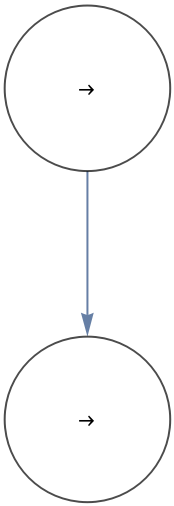### Options (1)

#### Verify (1)

 In:=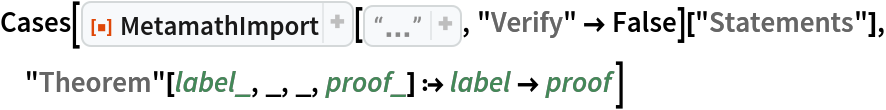Out=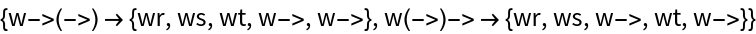In:=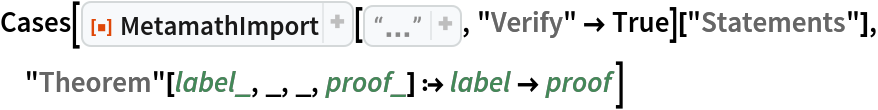Out=### Neat Examples (2)

Import the SetMM database:

 In:=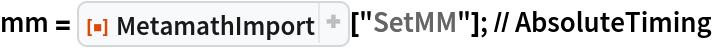Out=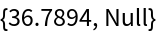In:=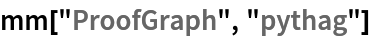Out=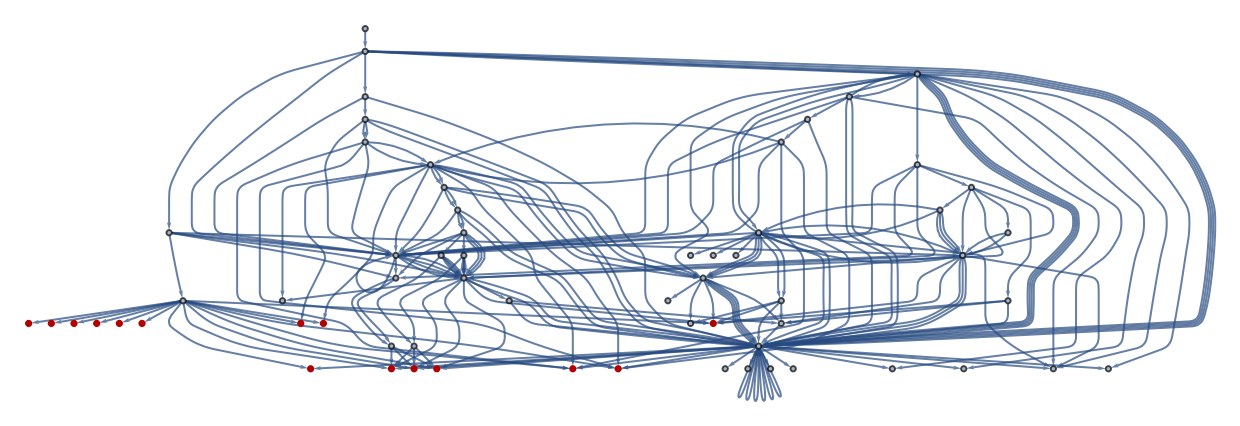In:=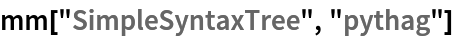Out=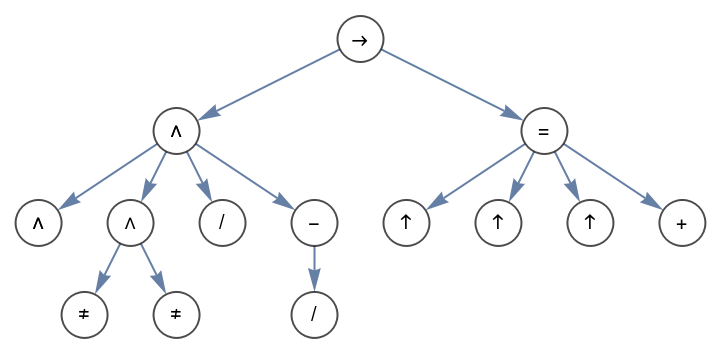In:=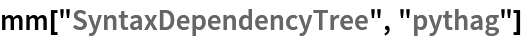Out=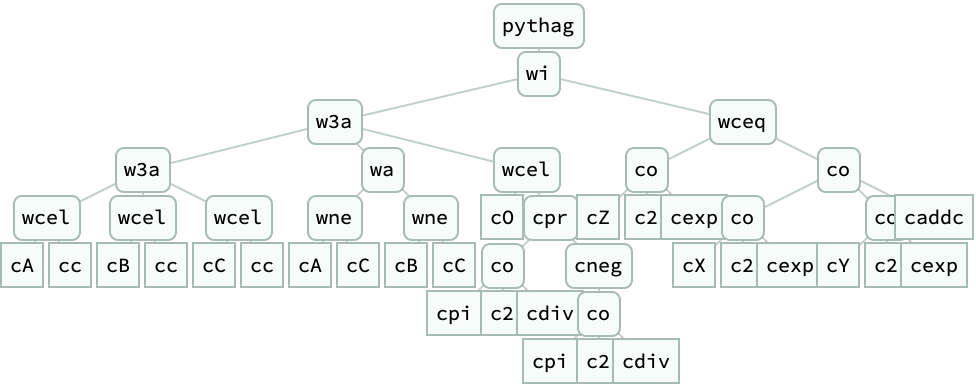In:=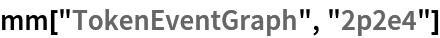Out=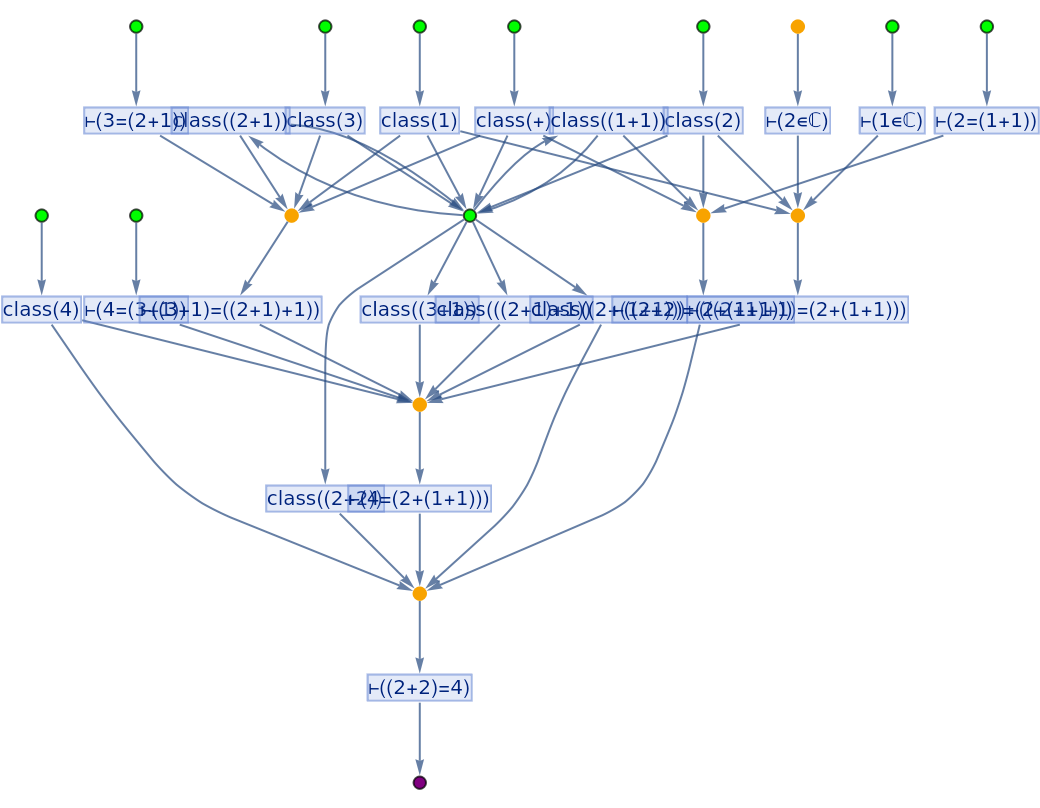Norm Megill's proofs of the Wolfram Axioms:

 In:=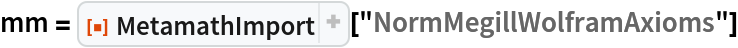Out=In:=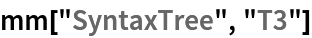Out=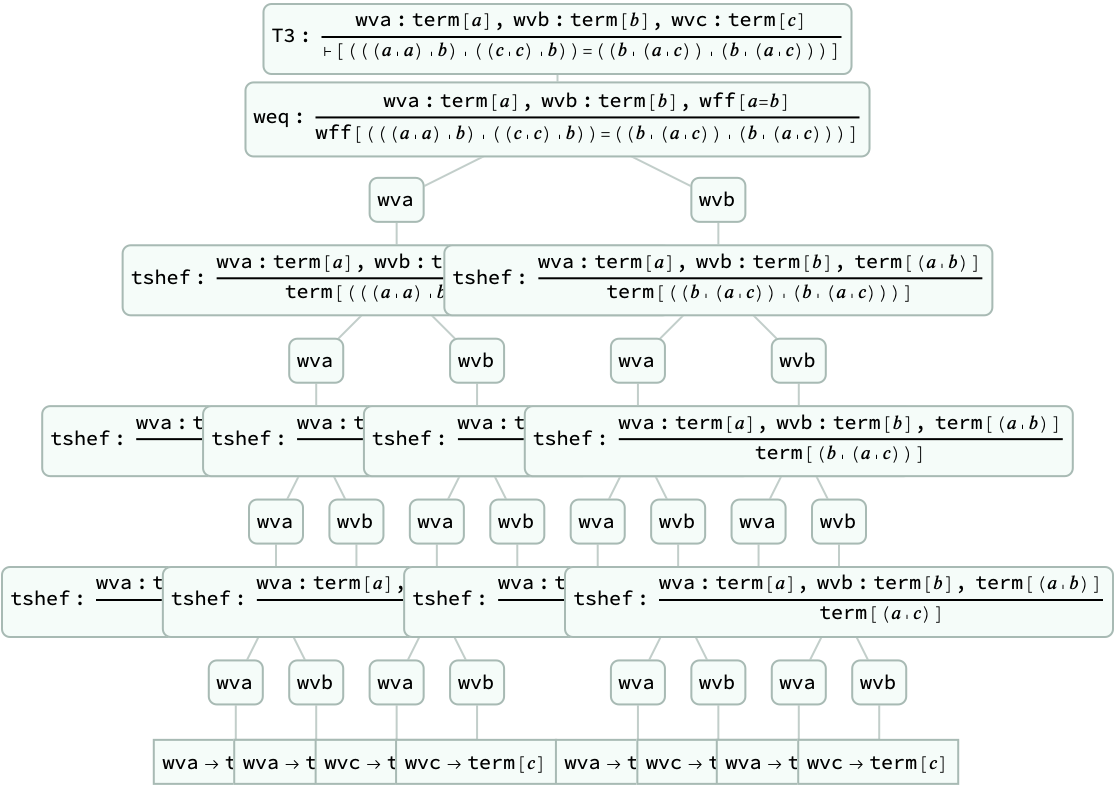In:=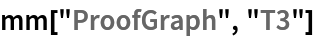Out=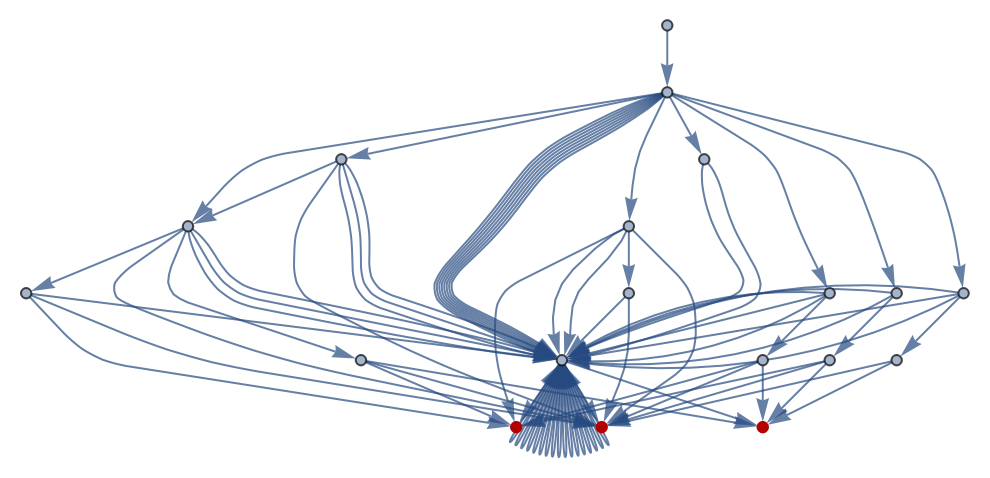In:=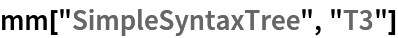Out=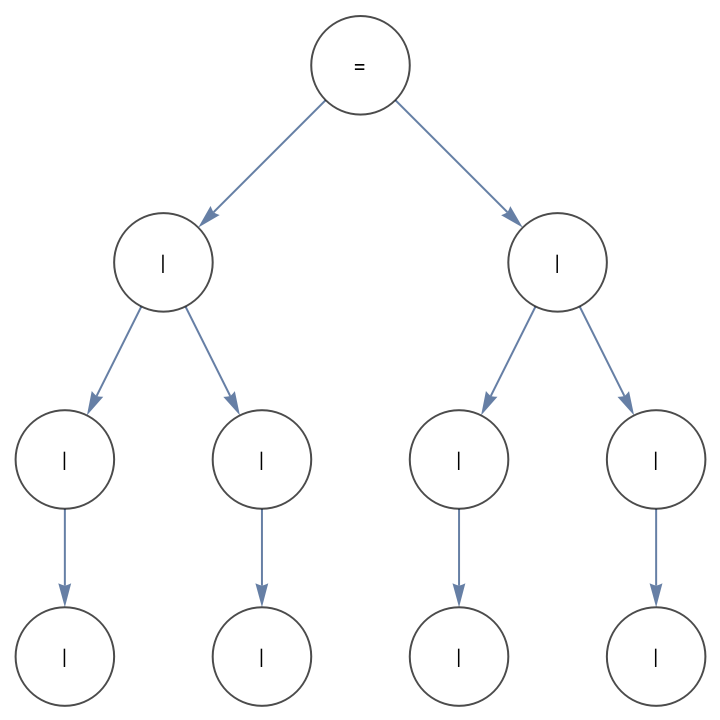N. Murzin

## Version History

• 2.0.0 – 07 March 2022
• 1.0.0 – 21 September 2020